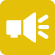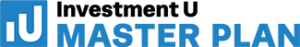## 1-Minute Survey

What Type of Investor Are You? – Take This 1-Min Survey to Find Out

Financial Literacy

# What Is an Annualized Return?

When investors seek to compare the performance of two assets over time, they should look at annualized return. Why this metric, specifically? Because it takes into account asset performance, time and compounding – the most important factors contributing to performance. Annualized return is the yearly rate of return for a given asset over a defined period of time. It is also known as the compounded annual growth rate (CAGR).

Investors who understand the meaning of annualized return and what it represents will find themselves better able to track the performance of assets against each other. It’s a metric that’s not difficult to calculate yet yields tremendous insight.## How to Calculate Annualized Return

The formulation for annualized return takes into account the annual return for each year over a defined number of years. There are really only two variables required: time and rate of return. The formula for annualized return is…

Annualized Return = ((1 + r1) × (1 + r2) × (1 + r3) × (1 + rn)(1/n)) – 1

or

Annualized Return = ((Ending value / Beginning value) ^ (1/n)) – 1

• r = rate of return
• n = number of periods

Because these formulas account for the total annual return of each period (year), this calculation also includes interest, dividends, capital gains and distributions. The result is a figure that shows the past performance of the asset based on the number of years held. It’s inclusive to the total return for each year, taking into account compounding for each year held.

## Comparing Rate of Return Over Different Time Periods

The best application of annualized return is comparing the performance of two funds over different time periods. This is helpful for investors who make investments at different times but want to compare the performance of those investments on a level playing field.

For example, Fund A might have a two-year total return of 15%, while Fund B might have a total return of 25% over four years. Looking at it from an annualized return standpoint takes into account the difference in performance based on the number of years. Annualized, the return of Fund A becomes 7.2%, while the return of Fund B becomes 5.7%.

Since time is one of the most important variables for investors to consider, annualized return is a more accurate form of assessment than other metrics that look only at cumulative return.

## Geometric vs. Arithmetic Mean

The reason annualized return is so useful is because it represents a geometric mean, as opposed to an arithmetic one. A geometric mean takes into account compounding; an arithmetic mean doesn’t. In practice, this affects the rate of return on an investment. For example…

• Over five years, Fund A has returns of 1%, 9%, 2%, 8% and 12%.
• Over five years, Fund B has returns of 5%, 6%, 7%, 8% and 6%.

The arithmetic mean of both is 6.4%. However, their geometric means differ. Fund A has a geometric mean of 5.93%; Fund B has a geometric mean of 6.4%. While this deviation might seem insignificant, such discrepancies can add up over time to huge gains (or losses). Measuring the arithmetic mean simply won’t give you the same insights as measuring the geometric mean. This is why annualized return is such a great metric – it accounts for compounding.

## Annualized Return vs. Average Return

Many investors confuse annualized return with average return. The two are very different concepts. Annualized return takes into account compounding; average return doesn’t. A simple example would be an investor who loses 50% one year and gains 100% the following year. Average return says the investor has a 25% rate of return, although it’s immediately evident to any investor that the annualized return is 0%.

## Pitfalls of Annualized Returns

Annualized return is evaluative, not predictive. It’s used to compare the past performance of different funds, not to predict their future performance. The future is a constant unknown. As such, it’s important to look at the overall volatility of any funds you’re comparing. Annualized returns can mask volatility and show a single appealing figure. If you’re not a fan of cyclical investments that rise and fall in varying degrees of volatility, you’ll need to probe deeper.

It’s also important to understand that the annualized return of mixed assets isn’t always a good indicator of their performance. Different types of assets perform differently within a portfolio, which makes comparing like-kind investments better than comparing clustered assets. Weighing the return of an individual stock against a highly leveraged ETF, for example, provides little more than surface insights for general comparison. Context matters.

Finally, annualized return is a linear measurement. Because it accounts for compounding, you can’t pick nonsequential years or compile outliers when tabulating this figure. Select a sequential range and use figures from consecutive periods to calculate annualized return.

## How to Use Annualized Return Effectively

Annualized return is an effective metric for comparing the past performance of like-kind investments over different time periods. For example, you might compare the return of Fund X over the past five years to the return of Fund Z over the last seven years. If you hold both investments, it’s important to understand their individual performances and contributions to your portfolio.

In fact, retirees use annualized returns to determine the performance of their assets. To stay up to date with the latest retirement strategies, sign up for the Wealthy Retirement e-letter below.

Use annualized return to better-understand the winners and losers in your portfolio and what’s raising vs. lowering your overall real rate of return. It’s a powerful metric to have when making decisions about how to weigh your portfolio, where to rebalance and how to evaluate other assets by comparison.

###### Articles by Leanna Kelly